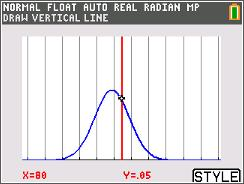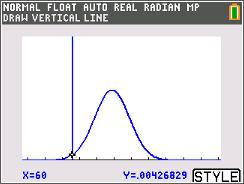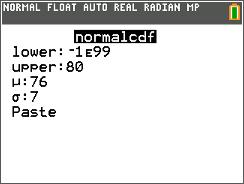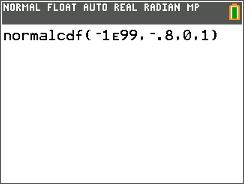••• ##### Device
• TI-84 Plus
• TI-84 Plus Silver Edition
•TI-84 Plus C Silver Edition
•TI-84 Plus CE
• ##### Report an Issue

Statistics: Areas In Intervals
by Texas Instruments#### Overview

Students estimate and find a given area under a normal curve.

#### Key Steps

•In the first problem, students will find the proportion of test scores that are less than or equal to a given score. After finding the proportion for two different given scores, students will find the proportion between those two scores. They will make an estimate from looking at the normal distribution curve and then find the actual answer using the normalcdf command.

•Then students will determine the proportion of weights greater than a given weight by finding the proportion less than or equal to the given weight and then subtract from 1.

•Students are introduced to the concept z-scores and using the standard normal distribution to find proportions. They will also find a test score given a z-score.